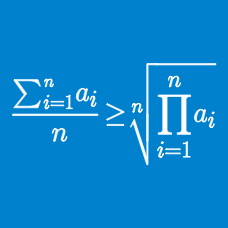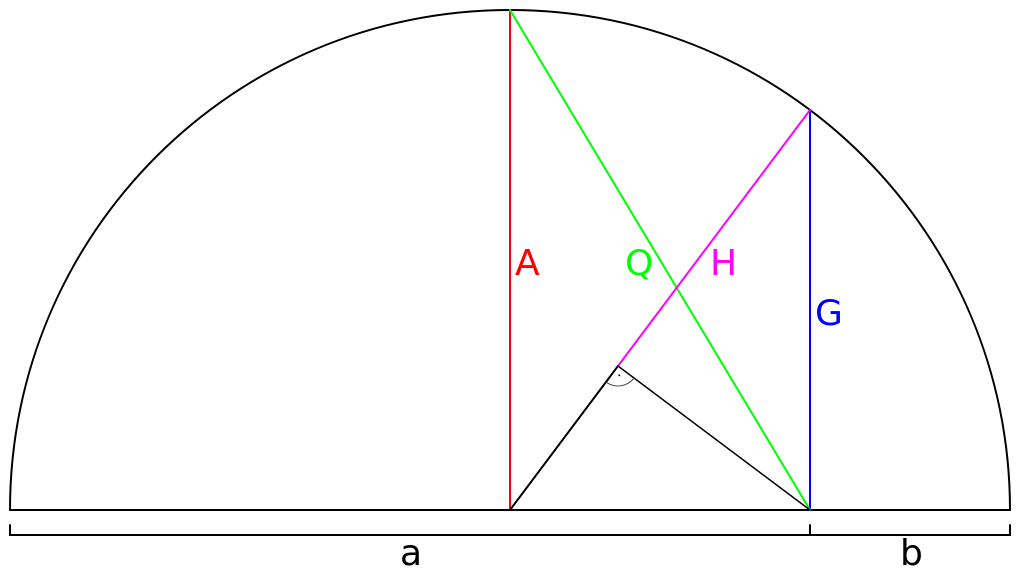Algebra

# Power Mean InequalitiesThe arithmetic mean $A = \frac{a+b}{2}$ and the geometric mean $G = \sqrt{ab}$ of non-negative quantities $a$ and $b$ are shown. Based on the diagram, which inequality is correct?

If the sum of two positive numbers is 18, what is the largest possible value of their product?

Hint. For non-negative numbers $a$ and $b,$ the Arithmetic Mean - Geometric Mean Inequality implies that $\frac{a+b}{2} \geq \sqrt{ab},$ and $\frac{a+b}{2} = \sqrt{ab} \text{ when } a = b.$

For values of $x$ such that $(8 +x)$ and $(1 -x)$ are positive, what is the maximum possible value of

$(8 +x)(1-x)?$

Hint. Apply the Arithmetic Mean - Geometric Mean Inequality.

If the sum of two legs of a right triangle is 20, what is the smallest possible value of the hypotenuse?

Hint. The quadratic mean, or root-mean-square of non-negative numbers $a$ and $b$ is greater than or equal to their arithmetic mean, i.e.

$\sqrt{\frac{a^2+b^2}{2}} \geq \frac{a+b}{2},$

with equality happening when $a = b.$

What is the quadratic mean of the four values $0, 0, 0,$ and $8?$

Note. The quadratic mean, or root-mean-square, of non-negative numbers $x_1, \ldots, x_n$ is

$\sqrt{\frac{x_1^2+\cdots + x_n^2}{n}}.$

×